# Tag: geometry

The previous post can be found [here].
Pierre Gabriel invented a lot of new notation (see his book [Representations of finite dimensional algebras] for a rather extreme case) and is responsible for calling a directed graph a quiver. For example,

$\xymatrix{\vtx{} \ar@/^/[rr] & & \vtx{} \ar@(u,ur) \ar@(d,dr) \ar@/^/[ll]}$

is a quiver. Note than it is allowed to have multiple arrows between vertices, as well as loops in vertices. For us it will be important that a quiver $Q$ depicts how to compute in a certain non-commutative algebra : the path algebra $\mathbb{C} Q$. If the quiver has $k$ vertices and $l$ arrows (including loops) then the path algebra $\mathbb{C} Q$ is a subalgebra of the full $k \times k$ matrix-algebra over the free algebra in $l$ non-commuting variables

$\mathbb{C} Q \subset M_k(\mathbb{C} \langle x_1,\ldots,x_l \rangle)$

Under this map, a vertex $v_i$ is mapped to the basis $i$-th diagonal matrix-idempotent and an arrow

$\xymatrix{\vtx{v_i} \ar[rr]^{x_a} & & \vtx{v_j}}$

is mapped to the matrix having all its entries zero except the $(j,i)$-entry which is equal to $x_a$. That is, in our main example

$\xymatrix{\vtx{e} \ar@/^/[rr]^a & & \vtx{f} \ar@(u,ur)^x \ar@(d,dr)_y \ar@/^/[ll]^b}$

the corresponding path algebra is the subalgebra of $M_2(\mathbb{C} \langle a,b,x,y \rangle)$ generated by the matrices

$e \mapsto \begin{bmatrix} 1 & 0 \\ 0 & 0 \end{bmatrix}$ $f \mapsto \begin{bmatrix} 0 & 0 \\ 0 & 1 \end{bmatrix}$

$a \mapsto \begin{bmatrix} 0 & 0 \\ a & 0 \end{bmatrix}$ $b \mapsto \begin{bmatrix} 0 & b \\ 0 & 0 \end{bmatrix}$

$x \mapsto \begin{bmatrix} 0 & 0 \\ 0 & x \end{bmatrix}$ $y \mapsto \begin{bmatrix} 0 & 0 \\ 0 & y \end{bmatrix}$

The name \’path algebra\’ comes from the fact that the subspace of $\mathbb{C} Q$ at the $(j,i)$-place is the vectorspace spanned by all paths in the quiver starting at vertex $v_i$ and ending in vertex $v_j$. For an easier and concrete example of a path algebra. consider the quiver

$\xymatrix{\vtx{e} \ar[rr]^a & & \vtx{f} \ar@(ur,dr)^x}$

and verify that in this case, the path algebra is just

$\mathbb{C} Q = \begin{bmatrix} \mathbb{C} & 0 \\ \mathbb{C}[x]a & \mathbb{C}[x] \end{bmatrix}$

Observe that we write and read paths in the quiver from right to left. The reason for this strange convention is that later we will be interested in left-modules rather than right-modules. Right-minder people can go for the more natural left to right convention for writing paths.
Why are path algebras of quivers of interest in non-commutative geometry? Well, to begin they are examples of _formally smooth algebras_ (some say _quasi-free algebras_, I just call them _qurves_). These algebras were introduced and studied by Joachim Cuntz and Daniel Quillen and they are precisely the algebras allowing a good theory of non-commutative differential forms.
So you should think of formally smooth algebras as being non-commutative manifolds and under this analogy path algebras of quivers correspond to _affine spaces_. That is, one expects path algebras of quivers to turn up in two instances : (1) given a non-commutative manifold (aka formally smooth algebra) it must be \’embedded\’ in some non-commutative affine space (aka path algebra of a quiver) and (2) given a non-commutative manifold, the \’tangent spaces\’ should be determined by path algebras of quivers.
The first fact is easy enough to prove, every affine $\mathbb{C}$-algebra is an epimorphic image of a free algebra in say $l$ generators, which is just the path algebra of the _bouquet quiver_ having $l$ loops

$\xymatrix{\vtx{} \ar@(dl,l)^{x_1} \ar@(l,ul)^{x_2} \ar@(ur,r)^{x_i} \ar@(r,dr)^{x_l}}$

The second statement requires more work. For a first attempt to clarify this you can consult my preprint [Qurves and quivers] but I\’ll come back to this in another post. For now, just take my word for it : if formally smooth algebras are the non-commutative analogon of manifolds then path algebras of quivers are the non-commutative version of affine spaces!

: http://www.neverendingbooks.org/index.php?p=71
: http://www.booxtra.de/verteiler.asp?site=artikel.asp&wea=1070000&sh=homehome&artikelnummer=000000689724
: http://www.arxiv.org/abs/math.RA/0406618

Here’s a part of yesterday’s post by bitch ph.d. :

But first of all I have to figure out what the hell I’m going to teach my graduate students this semester, and really more to the point, what I am not going to bother to try to cram into this class just because it’s my first graduate class and I’m feeling like teaching everything I know in one semester is a realistic and desireable possibility. Yes! Here it all is! Everything I have ever learned! Thank you, and goodnight!

Ah, the perpetual motion machine of last-minute course planning, driven by ambition and sloth!.

I’ve had similar experiences, even with undergraduate courses (in Belgium there is no fixed curriculum so the person teaching the course is responsible for its contents). If you compare the stuff I hoped to teach when I started out with the courses I’ll be giving in a few weeks, you would be more than disappointed.
The first time I taught _differential geometry 1_ (a third year course) I did include in the syllabus everything needed to culminate in an outline of Donaldson’s result on exotic structures on $\mathbb{R}^4$ and Connes’ non-commutative GUT-model (If you want to have a good laugh, here is the set of notes). As far as I remember I got as far as classifying compact surfaces!
A similar story for the _Lie theory_ course. Until last year this was sort of an introduction to geometric invariant theory : quotient variety of conjugacy classes of matrices, moduli space of linear dynamical systems, Hilbert schemes and the classification of $GL_n$-representations (again, smile! here is the set of notes).
Compared to these (over)ambitious courses, next year’s courses are lazy sunday-afternoon walks! What made me change my mind? I learned the hard way something already known to the ancient Greeks : mathematics does not allow short-cuts, you cannot expect students to run before they can walk. Giving an over-ambitious course doesn’t offer the students a quicker road to research, but it may result in a burn-out before they get even started!

Now that the preparation for my undergraduate courses in the first semester is more or less finished, I can begin to think about the courses I’ll give this year in the master class
non-commutative geometry. For a change I’d like to introduce the main ideas and concepts by a very concrete example : Ginzburg’s coadjoint-orbit result for the Calogero-Moser space and its
relation to the classification of one-sided ideals in the first Weyl algebra. Not only will this example give me the opportunity to say things about formally smooth algebras, non-commutative
differential forms and even non-commutative symplectic geometry, but it also involves what some people prefer to call _non-commutative algebraic geometry_ (that is the study of graded Noetherian
rings having excellent homological properties) via the projective space associated to the homogenized Weyl algebra. Besides, I have some affinity with this example.

A long time ago I introduced
the moduli spaces for one-sided ideals in the Weyl algebra in Moduli spaces for right ideals of the Weyl algebra and when I was printing a _very_ preliminary version of Ginzburg’s paper
Non-commutative Symplectic Geometry, Quiver varieties, and Operads (probably because he send a preview to Yuri Berest and I was in contact with him at the time about the moduli spaces) the
idea hit me at the printer that the right way to look at the propblem was to consider the quiver

$\xymatrix{\vtx{} \ar@/^/[rr]^a & & \vtx{} \ar@(u,ur)^x \ar@(d,dr)_y \ar@/^/[ll]^b}$

which eventually led to my paper together with Raf Bocklandt Necklace Lie algebras and noncommutative symplectic geometry.

Apart from this papers I would like to explain the following
papers by illustrating them on the above example : Michail Kapranov Noncommutative geometry based on commutator expansions Maxim Kontsevich and Alex Rosenberg Noncommutative smooth
spaces
Yuri Berest and George Wilson Automorphisms and Ideals of the Weyl Algebra Yuri Berest and George Wilson Ideal Classes of the Weyl Algebra and Noncommutative Projective
Geometry
Travis Schedler A Hopf algebra quantizing a necklace Lie algebra canonically associated to a quiver and of course the seminal paper by Joachim Cuntz and Daniel Quillen on
quasi-free algebras and their non-commutative differential forms which, unfortunately, in not available online.

I plan to write a series of posts here on all this material but I will be very
your name and email is required to do so, you do not have to register and you can even put some latex-code in your post but such a posting will first have to viewed by me to avoid cluttering of
nonsense GIFs in my directories).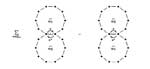Today Travis Schedler posted a nice paper on the arXiv
“A Hopf algebra quantizing a necklace Lie algebra
canonically associated to a quiver”
. I heard the first time about
necklace Lie algebras from Jacques Alev who had heard a talk by Kirillov
who constructed an infinite dimensional Lie algebra on the monomials in
two non-commuting variables X and Y (upto cyclic permutation of the
word, whence ‘necklace’). Later I learned that this Lie algebra was
defined by Maxim Kontsevich for the free algebra in an even number of
variables in his “Formal (non)commutative symplectic geometry” paper
(published in the Gelfand seminar proceedings 1993). Later I extended
this construction together with Raf Bocklandt in “Necklace Lie algebras and non-commutative symplectic
geometry”
. Here, the necklace Lie algebra appears from
(relative) non-commutative differential forms on a symmetric quiver and
its main purpose is to define invariant symplectic flows on quotient
varieties of representations of the quiver.
Travis Schedler
extends this construction in two important ways. First, he shows that
the Lie-algebra is really a Lie-bialgebra hence there is some sort of
group-like object acting on all the representation varieties. Even more
impoprtant, he is able to define a quantization of this structure
defining a Hopf algebra. In this quantization, necklaces play a role
similar to that of (projected) flat links in the plane whereas their
quantization (necklaces with a height) are similar to genuine links in
3-space.
Sadly, at the moment there is no known natural
representations for this Hopf algebra playing a similar role to the
quotient varieties of quiver-varieties in the case of the necklace Lie
bialgebra.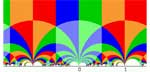Can
it be that one forgets an entire proof because the result doesn’t seem
important or relevant at the time? It seems the only logical explanation
for what happened last week. Raf Bocklandt asked me whether a
classification was known of all group algebras l G which are
noncommutative manifolds (that is, which are formally smooth a la Kontsevich-Rosenberg or, equivalently, quasi-free
a la Cuntz-Quillen). I said I didn’t know the answer and that it looked
like a difficult problem but at the same time it was entirely clear to
me how to attack this problem, even which book I needed to have a look
at to get started. And, indeed, after a visit to the library borrowing
Warren Dicks
lecture notes in mathematics 790 “Groups, trees and projective
modules” and browsing through it for a few minutes I had the rough
outline of the classification. As the proof is basicly a two-liner I
might as well sketch it here.
If l G is quasi-free it
must be hereditary so the augmentation ideal must be a projective
module. But Martin Dunwoody proved that this is equivalent to
G being a group acting on a (usually infinite) tree with finite
group vertex-stabilizers all of its orders being invertible in the
basefield l. Hence, by Bass-Serre theory G is the
fundamental group of a graph of finite groups (all orders being units in
l) and using this structural result it is then not difficult to
show that the group algebra l G does indeed have the lifting
property for morphisms modulo nilpotent ideals and hence is
quasi-free.
If l has characteristic zero (hence the
extra order conditions are void) one can invoke a result of Karrass
saying that quasi-freeness of l G is equivalent to G being
virtually free (that is, G has a free subgroup of finite
index). There are many interesting examples of virtually free groups.
One source are the discrete subgroups commensurable with SL(2,Z)
(among which all groups appearing in monstrous moonshine), another
source comes from the classification of rank two vectorbundles over
projective smooth curves over finite fields (see the later chapters of
Serre’s Trees). So
one can use non-commutative geometry to study the finite dimensional
representations of virtually free groups generalizing the approach with
Jan Adriaenssens in Non-commutative covers and the modular group (btw.
Jan claims that a revision of this paper will be available soon).
In order to avoid that I forget all of this once again, I’ve
written over the last couple of days a short note explaining what I know
of representations of virtually free groups (or more generally of
fundamental algebras of finite graphs of separable
l-algebras). I may (or may not) post this note on the arXiv in
the coming weeks. But, if you have a reason to be interested in this,
send me an email and I’ll send you a sneak preview.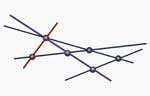After yesterday’s post I had to explain today what
point-modules and line-modules are and that one can really
describe them as points in a (commutative) variety. Seemingly, the
present focus on categorical methods scares possibly interested students
away and none of them seems to know that this non-commutative projective
algebraic geometry once dealt with very concrete examples.
Let
us fix the setting : A will be a quadratic algebra, that is, A is
a positively graded algebra, part of degree zero the basefield k,
generated by its homogeneous part A_1 of degree one (which we take to be
of k-dimension n 1) and with all defining relations quadratic in these
generators. Take m k-independent linear terms (that is, elements of A_1)
: l1,…,lm and consider the graded left A-module

L
= A/(Al1 + ... + Alm)

Clearly, the Hilbert series of this
module (that is, the formal power series in t with coefficient of t^a
the k-dimension of the homogeneous part of L of degree a) starts off
with

Hilb(L,t) = 1  + (n+1-m) t  + ...

and
we call L a linear d-dimensional module if the Hilbert series is
the power series expansion of

1/(1-t)^{d +1} = 1  + (d+1)t   +(d
+1)(d +2)/2 t^2   ... 

In particular, if d=0 (that is, m=n) then L
is said to be a point-module and if d=1 (that is, m=n-1) then L
is said to be a line-module. To a d-dimensional linear module L
one can associate a d-dimensional linear subspace of ordinary (that is,
commutative) projective n-space P^n. To do this, identify

P^n
= P(A 1^*)

the projective space of the n 1 dimensional space of
linear functions on the homogeneous part of degree one. Then each of the
linear elements li determines a hyperplane V(li) in P^n and the
intersection of the m hyperplanes V(l1),…,V(lm) is the wanted
subspace. In particular, to a point-module corresponds a point in
P^n and to a line-module a line in P^n. So, where
is the non-commutativity of A hidden? Well, if P is a point-module

P
= P0  + P1 +  P2   +... 

(with all components P_a one dimensional)
then the twisted module

P' = P1 +  P2  + P3  + ...

is
again a point-module and the map P–>P’ defines an automorphism on the
point variety. In low dimensions, it is often possible to
reconstruct A from the point-variety and automorphism. In higher
dimensions, one has to consider also the higher dimensional linear
modules.
When I explained all this (far clumsier as it was a
long time since I worked with this) I was asked for an elementary text
on all this. ‘Why hasn’t anybody written a book on all this?’ Well,
Paul Smith wrote such a book so have a look at his
homepage. But then, it turned out that the version one can download from
one of his course pages is a more recent and a lot more
categorical version. There is no more sight of a useful book on
non-commutative projective spaces and their linear modules which might
give starting students an interesting way to learn some non-commutative
algebra and the beginnings of algebraic geometry (commutative and
non-commutative). So, hopefully Paul still has the old version around
and will make it available… The only webpage on this I could find in
short time are the slides of a talk by Michaela Vancliff.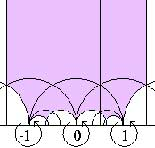Rather than going to the NOG
III Workshop
I think it is more fun to give a talk for the Capita
Selecta
-course for 2nd year students on “Monstrous Moonshine”. If
I manage to explain to them at least something, I think I am in good
shape for next year\’s Baby Geometry (first year) course. Besides,
afterwards I may decide to give some details of Borcherds\’ solution next year in my 3rd year
Geometry-course…(but this may just be a little bit
over-optimistic).
Anyway, this is what I plan to do in my
lecture : explain both sides of the McKay-observation
that

196 884 = 196 883 + 1

that is, I\’ll give
the action of the modular group on the upper-half plane and prove that
its fundamental domain is just C using the modular j-function (left hand
side) and sketch the importance of the Monster group and its
representation theory (right hand side). Then I\’ll mention Ogg\’s
observation that the only subgroups Gamma(0,p)+ of SL(2,Z)
for which the fundamental domain has genus zero are the prime divisors
p of teh order of the Monster and I\’ll come to moonshine
conjecture of Conway and Norton (for those students who did hear my talk
on Antwerp sprouts, yes both Conway and Simon Norton (via his
SNORT-go) did appear there too…) and if time allows it, I\’ll sketch
the main idea of the proof. Fortunately, Richard Borcherds has written
some excellent expository papers I can use (see his papers-page and I also discovered a beautiful
moonshine-page by Helena Verrill which will make my job a lot
easier.
Btw. yesterday\’s Monster was taken from her other monster story…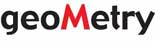One
of the best collections of links to homepages of people working in
non-commutative algebra and/or geometry is maintained by Paul Smith. At regular intervals I use it to check
up on some people, usually in vain as nobody seems to update their
homepage… So, today I wrote a simple spider to check for updates in
this list. The idea is simple : it tries to get the link (and when this
fails it reports that the link seems to be broken), it saves a text-copy
of the page (using lynx) on disc which it will check on a future
check-up for changes with diff. Btw. for OS X-people I got
lynx from the Fink Project. It then collects all data (broken
links, time of last visit and time of last change and recent updates) in
RSS-feeds for which an HTML-version is maintained at the geoMetry-site, again
using server side includes. If you see a 1970-date this means that I
have never detected a change since I let this spider loose (today).
Also, the list of pages is not alphabetic, even to me it is a surprise
how the next list will look. As I check for changes with diff the
claimed number of changed lines is by far accurate (the total of lines
from the first change made to the end of the file might be a better
approximation of reality… I will change this soon).
Clearly,
all of this is still experimental so please give me feedback if you
notice something wrong with these lists. Also I plan to extend this list
substantially over the next weeks (for example, Paul Smith himself is
not present in his own list…). So, if you want your pages to be
included, let me know at lieven.lebruyn@ua.ac.be.
For those on Paul\’s list, if you looked at your log-files today
you may have noticed a lot of traffic from www.matrix.ua.ac.be as
I was testing the script. I\’ll keep my further visits down to once a
day, at most…I
just finished the formal lecture-part of the course Projects in
non-commutative geometry
(btw. I am completely exhausted after this
afternoon\’s session but hopeful that some students actually may do
something with my crazy ideas), springtime seems to have arrived and
next week the easter-vacation starts so it may be time to have some fun
like making a new webpage (yes, again…). At the moment the main
matrix.ua.ac.be page is not really up to standards
and Raf and Hans will be using it soon for the information about the
Liegrits-project (at the moment they just have a beautiful logo). My aim is to make the main page to be the
starting page of the geoMetry site
(guess what M stands for ?) on which I want
to collect as much information as possible on non-commutative geometry.
To get at that info I plan to set some spiders or bots or
scrapers loose on the web (this is just an excuse to force myself
to learn Perl). But it seems one has to follow strict ethical guidelines
in doing so. One of the first sites I want to spider is clearly the arXiv but they have
a scary Robots Beware page! I don\’t know whether their
robots.txt file will allow me to get at any of
their goodies. In a robots.txt file the webmaster can put the
directories on his/her site which are off limits to robots and as I
don\’t want to do anything that may cause that the arXiv is no longer
available to me (or even worse, to the whole department) I better follow
these guidelines. First site on my list to study tomorrow will be The
Web Robots Pages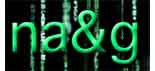Tomorrow
in our masterclass. The idea of this course (and its companion
Projects in non-commutative algebra run by Fred Van Oystaeyen) is
that students should make a small (original if possible) work, that may
At this moment the students
have seen the following : definition and examples of quasi-free algebras
(aka formally smooth algebras, non-commutative curves), their
representation varieties, their connected component semigroup and the
Euler-form on it. Last week, Markus Reineke used all this in his mini-course
Rational points of varieties associated to quasi-free
algebras
. In it, Markus gave a method to compute (at least in
principle) the number of points of the non-commutative Hilbert
scheme
and the varieties of simple representations over a
finite field. Here, in principle means that Markus demands a lot of
knowledge in advance : the number of points of all connected components
of all representation schemes of the algebra as well as of its scalar
extensions over finite field extensions, together with the action of the
Galois group on them … Sadly, I do not know too many examples were all
this information is known (apart from path algebras of quivers).
Therefore, it seems like a good idea to run through Markus’
calculations in some specific examples were I think one can get all this
: free products of semi-simple algebras. The motivating examples
being the groupalgebra of the (projective) modular group
PSL(2,Z) = Z(2) * Z(3) and the free matrix-products $M(n,F_q) * M(m,F_q)$. I will explain how one begins to compute things in these
examples and will also explain how to get the One
quiver to rule them all
in these cases. It would be interesting to
compare the calculations we will find with those corresponding to the
path algebra of this one quiver.
As Markus set the good
example of writing out his notes and posting them, I will try to do the
same for my previous two sessions on quasi-free algebras over the next
couple of weeks.